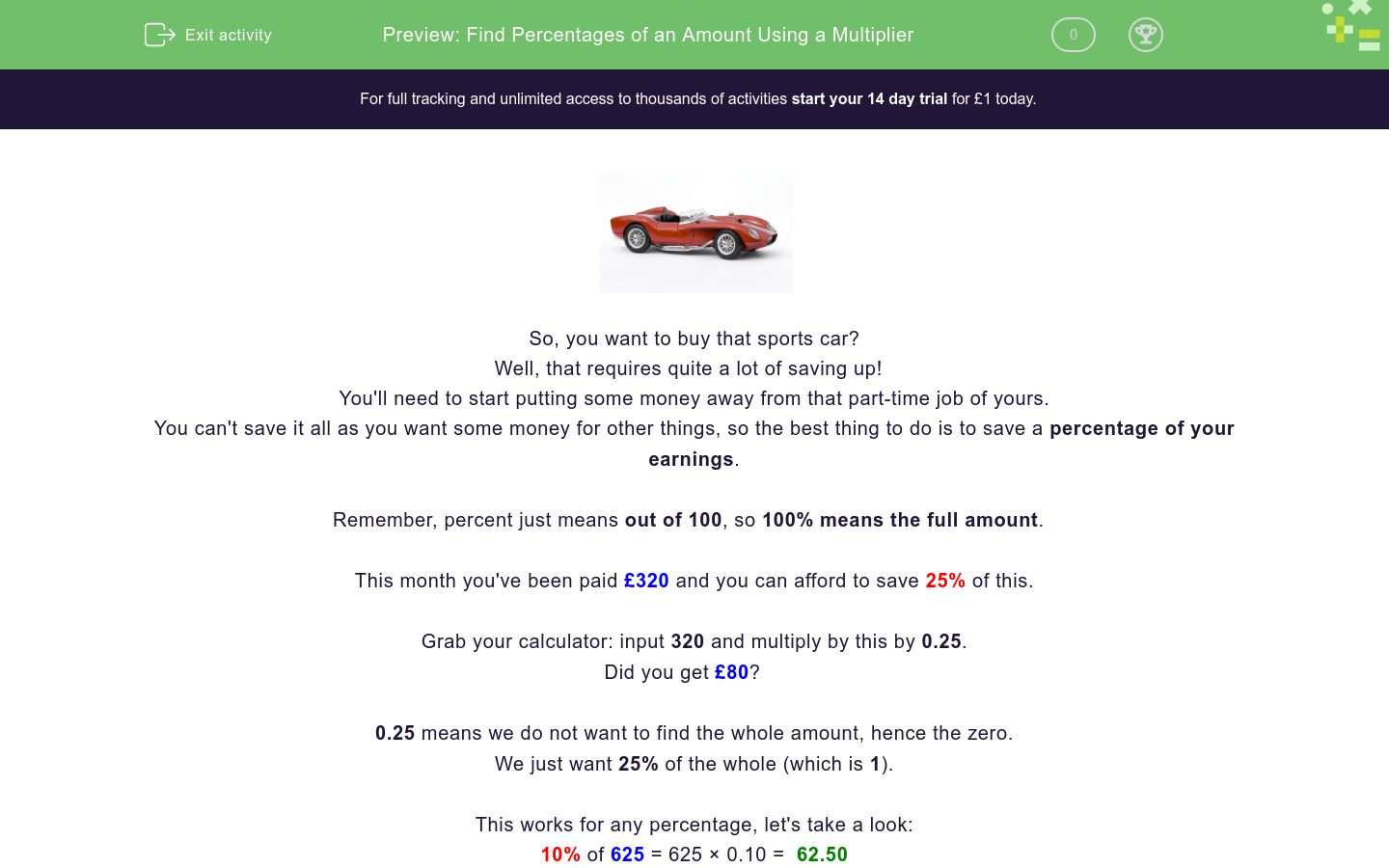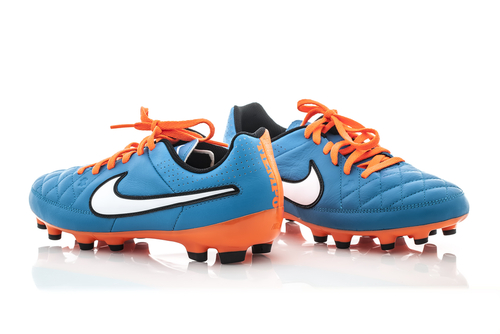# Find Percentages of an Amount Using a Multiplier

In this worksheet, students will find percentages of any given amount by multiplying using a decimal.Key stage:  KS 4

GCSE Subjects:   Maths

GCSE Boards:   AQA, Eduqas, Pearson Edexcel, OCR

Curriculum topic:   Ratio, Proportion and Rates of Change, Fractions, Decimals and Percentages

Curriculum subtopic:   Ratio, Proportion and Rates of Change, Percentages

Difficulty level:### QUESTION 1 of 10So, you want to buy that sports car?

Well, that requires quite a lot of saving up!

You'll need to start putting some money away from that part-time job of yours.

You can't save it all as you want some money for other things, so the best thing to do is to save a percentage of your earnings.

Remember, percent just means out of 100, so 100% means the full amount.

This month you've been paid £320 and you can afford to save 25% of this.

Grab your calculator: input 320 and multiply by this by 0.25.

Did you get £80?

0.25 means we do not want to find the whole amount, hence the zero.

We just want 25% of the whole (which is 1).

This works for any percentage, let's take a look:

10% of 625 = 625 × 0.10 =  62.50

15% of 328 = 15 × 0.15 = 49.40

53% of 497  = 497 × 0.53 = 263.41Take note:

When finding a percentage that is less than 10, you can use multipliers such as 1% = 0.01,  2% = 0.02, 3% = 0.03, etc.

When finding a percentage that is over 10, such as 17.5%, you should use the multiplier 0.175.

In this activity, we will find percentages of amounts by multiplying using a decimal.

You will want to have a calculator handy to help you, as these questions are designed to test your understanding of this process, not your mental maths.

Match each calculation below to its accurate answer or amount.

## Column B

12% of 52
19.78
23% of 86
10.08
36% of 72
6.24
18% of 56
25.92

Here's another one for you to work out.

Can you match each calculation below to its accurate amount?

## Column B

7% of 93
0.09
1% of 9
6.51
9% of 165
0.32
2% of 16
14.85

What is 30% of £753 added to 43% of £421?

## Column B

7% of 93
0.09
1% of 9
6.51
9% of 165
0.32
2% of 16
14.85The latest football boots are on sale.

They originally cost £128

Billy wants to buy them, and the sale states that there is 33% off.

How much does Billy save?

£42.22

£42.28

£42.24

£42.25An ice cream label lists the contents as containing 12.5% fat.

How much fat is there in a 750 g ice cream?

£42.22

£42.28

£42.24

£42.25The population of Spain is 46.72 million people.

43.5% of the population travel by train at least once a week.

How many people is this?

20.3232

20,323,200

20,323

2,032.32Would you rather be attacked by 35% of 120 lions or 20% of 150 lions?

35% of 120 lions

20% of 150 lions

They are both the same so either

Sarah says that 8% of 542 is 433.60, but Derek says that the answer is 43.36.

Who is correct?

Sarah

Derek

Neither of themMrs. Jones has just moved house and needs to allocate a percentage of her salary to pay her bills.

Mrs. Jones earns £1600 per month.

Calculate the amounts for each of her bills listed below then type your answer into the correct space using only numbers.

Sarah

Derek

Neither of them

What is the difference between 33.3% of £5,465 and 22.2% of £4,576?

Sarah

Derek

Neither of them

• Question 1

Match each calculation below to its accurate answer or amount.

## Column B

12% of 52
6.24
23% of 86
19.78
36% of 72
25.92
18% of 56
10.08
EDDIE SAYS
Did you apply the steps from our Introduction accurately? Hopefully you remembered to use the multipliers (0.12, 0.23, 0.36 and 0.18) when calculating these. Let's look at the first question together: 12% of 52 We need to type in: 52 × 0.12 = 6.24 Can you follow this example to match the other pairs independently? Always check your calculator display to check you have entered the multiplier correctly before committing to your answer. It's so easy to forget to check!
• Question 2

Here's another one for you to work out.

Can you match each calculation below to its accurate amount?

## Column B

7% of 93
6.51
1% of 9
0.09
9% of 165
14.85
2% of 16
0.32
EDDIE SAYS
It's easy to freak out when you see a single percentage is required. Just remember that any percentage which is less than 10 needs that extra zero to make it work. So our multipliers here will be: 0.07, 0.01, 0.09 and 0.02. Let's look at one example together: 7% of 93 We need to type in: 93 × 0.07 = 6.51 Can you follow this example to match the other pairs independently? Remember that 0.2 means 20%, whereas 0.02 means 2% - these are quite different!
• Question 3

What is 30% of £753 added to 43% of £421?

EDDIE SAYS
Here we need to find the first percentage, then find the second, and, finally, add them together. It is too easy to get into a mess by trying to hold a lot of information in your head so don't forget to make a note of each step! Your working should look something like this: 30% of £753 = 753 × 0.3 = 225.9 43% of £421 = 421 × 0.43 = 181.03 225.9 + 181.03 = £406.93 How did you get on with that one?
• Question 4The latest football boots are on sale.

They originally cost £128

Billy wants to buy them, and the sale states that there is 33% off.

How much does Billy save?

£42.24
EDDIE SAYS
This type of question could catch us out, but we are too wise for this. When you are working with money make sure your calculator is set to show answers with two decimal places. Here we need to type in: 128 × 0.33 = £42.24 128 × .33 could show as 42.2 if your calculator is not set correctly and then you would just be guessing the last digit, which is not good! Top tip: Use your own calculator in any exams as it will be familiar to you and you will hopefully have learnt how to adjust the settings to your preferences. You don't want to score any own goals!
• Question 5An ice cream label lists the contents as containing 12.5% fat.

How much fat is there in a 750 g ice cream?

EDDIE SAYS
This type of question can be confusing as there is a decimal point in the percentage. Even a gifted mathematician can only work with one decimal point at a time. When using the multiplier, ignore the decimal point from the percentage. Our calculation here needs to be: 750 × 0.125 = 93.75 Try putting 750 x 0.12.5 into your calculator as a test... see even the calculator gets confused with two decimal points!
• Question 6The population of Spain is 46.72 million people.

43.5% of the population travel by train at least once a week.

How many people is this?

20,323,200
EDDIE SAYS
Big numbers like this can drive you mad. They don't need to be complicated though! For this, if we type in 46.72 × 0.435, we would have got 20.3232. However, our calculator doesn't know we mean millions! We need to convert the original number into millions first, to reach: 46720,000 × 0.435 = 20,323,200 Around 20 million makes sense doesn't it, as 50% would be around 23 million?
• Question 7Would you rather be attacked by 35% of 120 lions or 20% of 150 lions?

20% of 150 lions
EDDIE SAYS
Quite often you could be asked to compare percentages. We need to calculate both options before we can give an informed answer. 35% of 120 = 120 × 0.35 = 42 20% of 150 = 150 × 0.2 = 30 So the lesser numbers of lions would be calculated from 20% of 150. Neither answer would be great for you though - they would win for sure!
• Question 8

Sarah says that 8% of 542 is 433.60, but Derek says that the answer is 43.36.

Who is correct?

Derek
EDDIE SAYS
542 × 0.08 = 43.36 So Derek is correct. Sarah has made the mistake of using the multiplier of 0.80 instead of 0.08. So she has found 80% rather than 8%. This is a mistake which is often made when working with percentages below 10%. Be vigilant about making this type of error yourself in this type of question - learn from Sarah's mistake!
• Question 9Mrs. Jones has just moved house and needs to allocate a percentage of her salary to pay her bills.

Mrs. Jones earns £1600 per month.

Calculate the amounts for each of her bills listed below then type your answer into the correct space using only numbers.

EDDIE SAYS
This is an example of how we can use our percentage skills in a problem solving question. We need to calculate each percentage first, then add them together, and finally subtract this total from her total earnings (£1600). Your working should look something like this: 24%: 1600 × 0.24 = 384 12.5%: 1600 × 0.125 = 200 19%: 1600 × 0.19 = 304 384 + 200 + 304 = 888 1600 - 888 = 712 It looks like she has enough left to buy herself something she wants or to save towards a bigger purchase like a car.
• Question 10

What is the difference between 33.3% of £5,465 and 22.2% of £4,576?

EDDIE SAYS
Again, don't let the decimal points in our percentage put you off here. 33.3% of £5465: 5465 × 0.333 = 1819.845 22.2% of £4576 = 4576 × 0.222 = 1015.872 'Find the difference' just means subtract one from the other: 1819.845 - 1015.872 = 803.973 We are talking about money here, so we need to round up to 2 decimal places, as we cannot have part of a penny. So our final answer is £803.97. Well done for completing this activity. That's percentage perfection!
---- OR ----

Sign up for a £1 trial so you can track and measure your child's progress on this activity.

### What is EdPlace?

We're your National Curriculum aligned online education content provider helping each child succeed in English, maths and science from year 1 to GCSE. With an EdPlace account you’ll be able to track and measure progress, helping each child achieve their best. We build confidence and attainment by personalising each child’s learning at a level that suits them.

Get started